Math Noodles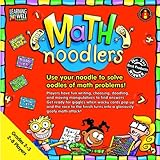Tri Facta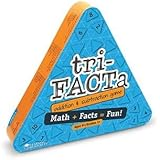Pizza Fraction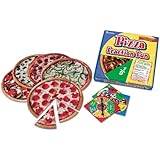Learning PalettesMultiplication or Addition Machine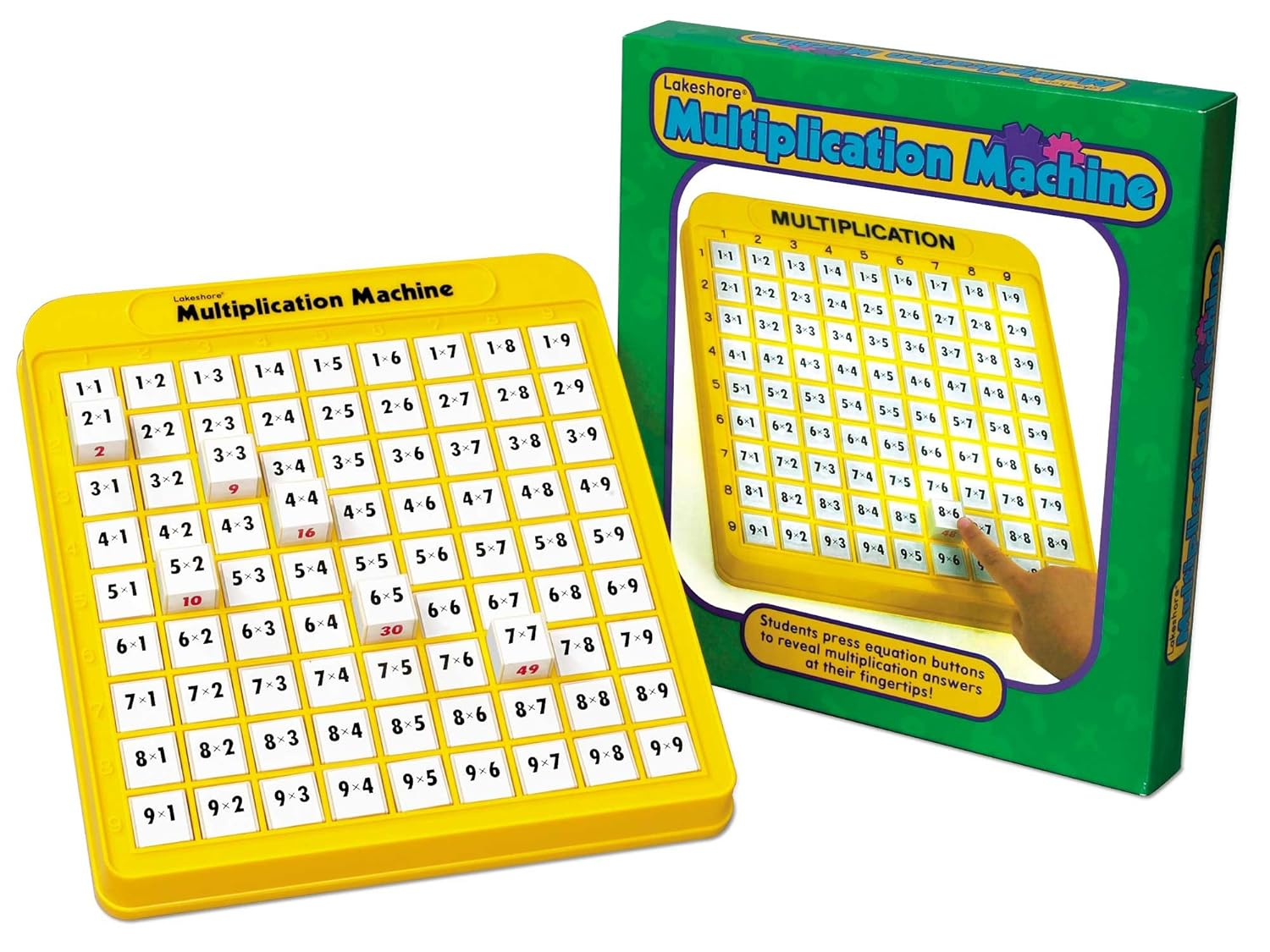Times Tales DVDThree Corner Flashcards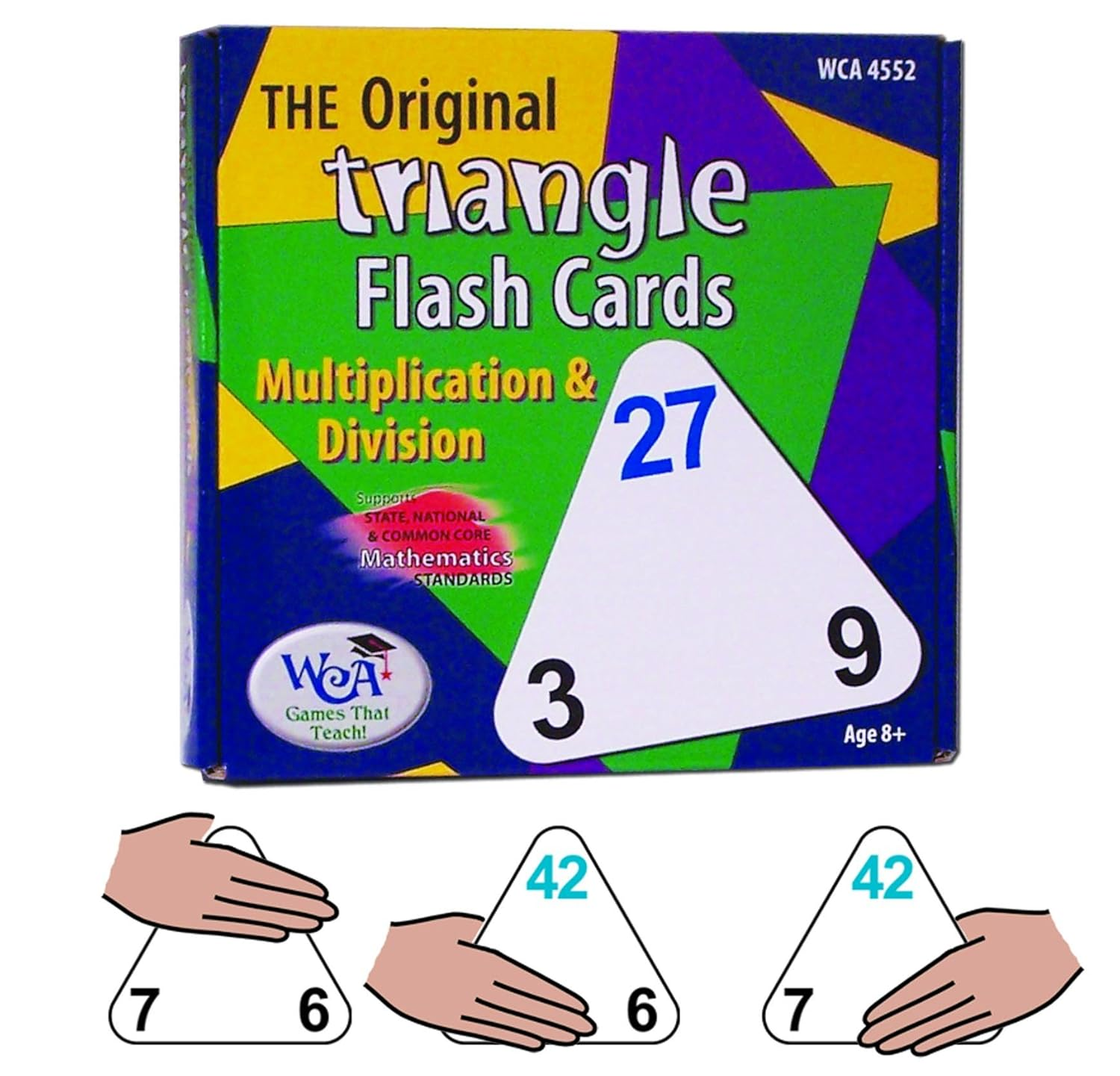Learning WrapsLife of FredThree-CornerThree-CornerMath ManipulativeSir CumferenceMathStartMango MathMango Math - Kindergarten

 Measurement Guess My Size Directly compare two objects with a measurable attribute in common to see which object has more/less. CCSS K.MD 2 Measurement Super Sandcastle Describe measureable attributes of objects, such as length. Describe several measurable attributes of a single object. CCSS K.MD 1 Algebraic Thinking Add'em Up Represent addition and subtraction with objects, mental images, drawings, verbal explanation, expression or equation. CCSS K.OA.1 Algebraic Thinking Azuki Addition For any number from 1 to 9, find the number that makes 10 when added to the given number. CCSS K.OA 4 Algebraic Thinking Clip On Fluently composing and decomposing numbers to 10; join sets of objects to show addition and subtraction. CCSS K.OA 1 & 3 Algebraic Thinking Fill the Chute Correlating the number rolled with number of tiles needed; gaining a foundation for place value. CCSS K.OA 2 Algebraic Thinking Nurtle the Turtle Decompose numbers less than or equal to 10 into pairs in more than one way and record each decomposition. CCSS K.OA 3 Numbers & Operations 20 Little Monkeys Compose and decompose number from 11 - 19 understanding that these number are composed of tens and ones. CCSS K.NBT 1 Numbers & Operations Domino Dash Understand that the last number name said tells the number of objects counted. The objects is the same regardless of their arrangement. CCSS K.CC 4b Numbers & Operations Domino Train Count to answer "how many" questions about as many as 20 things arranged in a line or rectangular array. Compare the amounts. CCSS K.CC 5 Numbers & Operations Follow Suit Understand that each successive number name refers to a quantity that is one larger. CCSS K.CC 4c Numbers & Operations Fruit Loot When counting objects, say the number names in the standard order, pairing each object with one and only one number name and each number name with one and only one object. CCSS K.CC 4b Numbers & Operations Garden Path Count forward and backward beginning from a given number within the known sequence. CCSS K.CC 2 Numbers & Operations Leap Frog Compare numbers between 1 & 30. CCSS K.CC 7 Use terms such as above, below. in front, behind and next to locate numbers on a number line. CCSS K.G 1 Numbers & Operations More or Less Identify whether the number of objects in one group is greater than, less than, or equal to the number of objects in another group. CCSS K.CC 6 Numbers & Operations Trailing Along Count forward beginning from a given number with the known sequence and write numbers. CCSS K.CC 2 Geometry Pattern Creator Correctly name shapes regardless of their orientations or overall size. CCSS K.G 6 Geometry Shape Search Describe objects in the environment using names of shapes. CCSS K.G 1 Ordering Data Button Bonanza Describe measurable attributes of objects. Describe several measurable attributes of a single object. CCSS K.MD 3 Ordering Data Wee Bears Classify objects into given categories; count the number of objects in each category and sort the categories by count. CCSS K.MD 3

Mango Math - First Grade

Measurement Door to Door Understand that the length measurement of an object is the number of same-size length units that span it with no gaps or overlays. CCSS 1.MD 2
Measurement Ms. Fox, What Time Is It Tell time in hours and half-hours using analog clocks. CCSS 1.MD 3
Algebraic Thinking Crack the Combo Using addition within 20 to solve word problems involving a situation of adding to, comparing. CCSS 1.OA
Algebraic Thinking Cue Apply properties of operations as strategies to add and subtract. CCSS 1.OA 3
Algebraic Thinking Double Down Add and subtract within 20, demonstrating fluency for addition and subtraction using strategies. CCSS 1.OA 6
Algebraic Thinking Funny Faces Relate counting to addition by counting on 2 to add to 2 or counting on 3 to add 3. CCSS 1.OA 5
Algebraic Thinking Golden Hexagon Use addition within 20 to solve word problems involving situations of adding to, putting together, and comparing with unknowns by using objects. CCSS 1.OA 1
Algebraic Thinking Hidden Link Relative counting to addition and subtraction (by counting on 2 or add 2) CCSS 1.OA 5
Algebraic Thinking Jump Off Use addition and subtraction with 30 to solve situations of adding to, taking from, and comparing using drawings. CCSS 1.OA 1
Algebraic Thinking Over the Rainbow Determine the unknown whole number in an addition or subtraction equation relating three whole numbers. CCSS 1.OA 8
Algebraic Thinking Salute Understand subtraction as an unknown-addend problem. CCSS 1.OA 4
Algebraic Thinking Seek and Hide Adding three numbers together working on Commutative & Associative property of addition. CCSS 1.OA 3
Numbers & Operations Going Up and Down Subtract multiples of 10 in the range of 10 - 90 from multiples of 10 in a range of 10 - 90. CCSS 1.NBT 6
Numbers & Operations Number Neighbors Given a two-digit number, mentally find 10 more or 10 less than the number, without having to count. CCSS 1.NBT 5
Numbers & Operations River Crossing Compare two-digit numbers based on meanings of the tens and ones digit, recording the results of comparisons with the symbols >, =, and <. CCSS 1.NBT 3
Numbers & Operations Roller Coaster Ride Add within 100, including adding a two-digit number and a one-digit number and adding a two-digit number and a multiple of ten. CCSS 1.NBT 4
Geometry 7 Magic Shapes Compose two-dimensional shapes to create a composite shape using; rectangles, squares, trapezoids, triangles. CCSS 1.G 2
Geometry Castle Creator Compose three-dimensional shapes to create a composite shape using; cubes, rectangular prisms, cones, cylinders. CCSS 1. G2
Ordering Data Blink Organize, represent and interpret data with up to three characteristics, number, shape, color. CCSS 1.MD 4
Ordering Data Stanley Cup Organize, represent, and interpret data with up to three categories; ask and answer questions about the total number of data points. CCSS 1.MD4

Mango Math - Second Grade

Measurement Broken Rules Estimate lengths using units of inches and centimeters. CCSS 2.MD 3
Measurement Coin Collecting Solve problems involving quarters, dimes, nickels, and pennies. CCSS. 2.MD 8
Measurement Make the Time Practicing telling time up to 5 minutes, comparing times and relating times to daily activities. CCSS 2. MD 7
Algebraic Thinking Buddy Up Determine whether a group of objects has an odd or even number of members by pairing objects or counting them by 2s. CCSS 2.OA 3
Algebraic Thinking Flip It Fluently add and subtract within 20 using mental strategies. CCSS 2.OA 2
Algebraic Thinking Fruit Salad Solve word problems involving adding to, taking from, comparing with unknowns in all positions. CCSS 2.OA 1
Algebraic Thinking Variable Veggies Use addition and subtraction within 100 to solve one-and two-step word problems involving adding to, taking from, comparing with unknowns in all positions. CCSS 2.OA 1
Numbers & Operations 4 Way Shuffle Add & subtract within 1000 using strategies & algorithms based on place value, properties of operations, & the relationship between addition & subtraction. CCSS 3.NBT 2
Numbers & Operations Big, Bigger, Biggest Read and write numbers to 1000 using base-ten numerals, number names and expanded form, comparing numbers CCSS 3NBT 3 & 4
Numbers & Operations Coyote Crazy Add up to four two-digit numbers using strategies based on place value and properties of operations. CCSS 2.NBT 6
Numbers & Operations Donut Whole Fluently add and subtract within 100 using strategies based on place value. CCSS 2.NBT 5
Numbers & Operations Lose Your Cents Understand subtraction of numbers based on hundreds, tens ones having to compose or decompose tens or hundreds. CCSS 2.NBT 7
Numbers & Operations Mango Math Compare two three-three digit numbers based on meanings of the hundreds, tens, and ones digits, using >, =. < symbols to record the results of comparisons. CCSS 2.NBT 4
Numbers & Operations Number Neighbors II Mentally add 10 or subtract 10 from a given number. CCSS 2.NBT 8
Numbers & Operations Push the Envelope Understand that two digits in a two-digit number represents amounts of hundreds, tens and ones. CCSS 1.NBT 2
Numbers & Operations Slip 'N Slide Fluently add and subtract within 100 using strategies based on place value and properties of operations. CCSS 2.NBT 5
Geometry 4 Squares Partition a rectangle into rows and columns of same-size squares and count to find total. CCSS 2.G2
Geometry Goody Grab Partition rectangles into equal shares, describe the shares using words like halves, thirds, fourths. Recognize equal shares of identical wholes. CCSS 2.G3
Ordering Data Double Digit Pig Collect data to gather information about the likeliness of something happening. Use data to make decisions. CCSS 2.MD 10
Ordering Data Worm Hole Generate measurement data by measuring lengths. Show the measurement by making bar graphs. CCSS 2.MD 9

Mango Math - Third Grade

Measurement Got Room Recognize area as an attribute of plane figures and understand concepts of area measurement CCSS 3MD 5
Measurement Measurement Mango Tree Generate measurement data by measuring lengths using ruler marked with halves and fourths of an inch. CCSS 3.MN 4
Measurement Race for Time Tell and write time to the nearest minute and measure time intervals in minutes. Solve word problems involving addition and subtraction of time intervals. CCSS 3MD1
Algebraic Thinking Array Around Use multiplication and division within 100 to solve problems in situations involving equal groups, arrays, & measurement quantities, by using drawings and equations. CCSS 3.OA 3 3.MD7a
Algebraic Thinking Crazy Cash Fluently add and subtract within 100 using strategies and algorithms based on place value, proprieties of operation and the relationship between addition and subtraction. CCSS 3.NBT 2
Algebraic Thinking Doorbell Rings Interpret whole-number quotients of whole numbers, interpret the number of objects in each share. CCSS 3.OA 2
Algebraic Thinking Feet on the Farm Use multiplication within 100 to solve word problems in situations involving equal groups by drawing and equations with a symbol for the unknown number. CCSS 3.OA 3
Algebraic Thinking Gumball Jumble Interpret whole-number quotients of whole numbers, interpret the number of objects in each share. CCSS 3.OA 2
Algebraic Thinking In & Out Burgers Determine the unknown whole number in a multiplication or division equation relating three whole numbers. CCSS 3.OA 4
Algebraic Thinking Salute Understand division as an unknown-factor problem. CCSS 3.OA 6
Numbers & Operations Balance Or Bust Fluently add and subtract within 1000 using strategies and algorithms based on place value CCSS 3NBT 2
Numbers & Operations Cookie Munch Understand a fraction 1/b as the quantity formed by 1 part when a whole is partitioned into b equal parts; understand a fraction a/b as the quantity formed by a parts of 1/b CCSS 3.NF
Numbers & Operations Spilt Milk Understand a fraction 1/b as the quantity formed by 1 part when a whole is partitioned into b equal parts; CCSS 3.NF 1 Measure liquids CCSS 3.MD2
Numbers & Operations Spiral Around Identify arithmetic patterns and explain them using properties of operations. CCSS 3.OA 9 & 3.NBT 3
Geometry Bug Zoo Solve real world and mathematical problems involving perimeters of polygons, including finding perimeter given the side lengths. CCSS 3.MD 8
Geometry Mystery Shape Understand that shapes in different categories may share attributes and that the shared attributes can define a larger category. CCSS 3.G 1
Geometry Polygon P.I. Recognize rhombus, rectangles and squares as examples of quadrilaterals. CCSS 3.G 1
Geometry Tile Shuffle Recognize area as an attribute of plane figures and understand concepts of area measurement. CCSS 3.MD 5
Ordering Data Candy Catastrophe Draw a scaled bar graph to represent a data set with several categories. CCSS 3.MD 3
Ordering Data What 2 Wear Draw a scaled bar graph to represent a data set with several categories. CCSS 3.MD 3

Mango Math - Fourth Grade

Measurement Check Out Challenge Use the addition and subtraction to solve word problems involving money including problems involving simple decimals. CCSS 4.MD 2
Measurement Measure for Measure Know relative sizes of measurement units within one system of units. Express measurements in a larger unit in terms of smaller unit. CCSS 4.MD 1
Measurement Wind Up Know relative size of measurement units within one system of units cm, m; in, ft.; record measurement equivalence. CCSS 4.MD 1
Algebraic Thinking Be Fair Multiply or divide to solve word problems involving multiplicative comparison, use symbol for the unknown number. CCSS 4.OA 2
Algebraic Thinking Dollar Dilemma Solve multistep word problems posed with whole numbers and having whole-number answers using the four operations. CCSS 4.OA 3
Algebraic Thinking Factor Game Find all factor pairs for a whole number in the range 1 - 100. Recognize multiple of each of its factors. CCSS 4.OA
Algebraic Thinking Jumping Around Generate a number that follows a given rule. Identify apparent features of the pattern that were not explicit in the rule itself. CCSS 4.OA 5
Algebraic Thinking Tic-Fact-Toe Find all the factor pairs for a whole number in the range 1 - 100. Recognize that a whole number is a multiple of each of its factors. CCSS 4.OA 4
Numbers & Operations Candy Caper Understand addition and subtraction of fractions as joining and separating parts referring to the same whole. CCSS 4NF 1
Numbers & Operations Dealing with Decimals Compare two decimals to hundredths by reasoning about their size. CCSS 4.NF 7
Numbers & Operations Frog Action Solve problems involving multiplication of a fraction by a whole number using visual fraction models. CCSS 4.NF 4c
Numbers & Operations Grab Bag Solve word problems involving addition and subtraction of fractions referring to the same whole and having like denominators. CCSS 4.NF 3d
Numbers & Operations Lattice Multiply Multiply a whole number of up to four digits by a one-digit whole number, & multiply 2 two-digit numbers, using strategies based on place value CCSS 4.NBT 4
Numbers & Operations Leftovers Find Whole-number quotients and remainders with up to 4-digit dividends & 1-digit divisors CCSS 4.NBT 6
Numbers & Operations Name that Card Compare two fractions with different numerators and different denominators. CCSS 4.NF 2
Numbers & Operations Speed Rounder Use place value understanding to round multi-digit whole numbers to any place. CCSS 4.NBT 3
Numbers & Operations Spin to Win Recognize that in a multi-digit whole number, a digit in one place represents 10 times what is represents in the place to the right. CCSS 4.NBT 1
Geometry Corralling Polygons Classify two-dimensional figures based on the presence or absence of angles, perpendicular and parallel lines CCSS 4.G 1 & 2
Geometry What's Your Angle Understand concepts of angle and measurement angle. CCSS 4.MD 5
Ordering Data Horse Race Make a line plot to display a data set of measurement in fractions of a unit. CCSS 4.MD 4

Mango Math - Fifth Grade

Measurement Liters, Meters, and Grams Convert among different-sized standard measurement units within a given measurement system (convert 5 cm to .05 m) CCSS 5.MD 1
Measurement Sugar Heights Measure volumes by counting unit cubes, using cubic cm. Relate volume to the operation of multiplication and addition and solve real world problems. CCSS 5.MD 3 & 4
Algebraic Thinking Cash Machine Generate numerical patterns using two given rules. Identify apparent relationships between terms. CCSS 5.OA 3
Algebraic Thinking Krypto Use parentheses, brackets, or braces to numerical expressions and evaluate expressions with these symbols. CCSS 5.OA 1
Algebraic Thinking Stick To It Generate numerical patterns using two given rules. Identify relationships between rules. CCSS 5.OA 3
Numbers & Operations Box It Up Add fractions with unlike denominators by replacing given fractions with equivalent fractions. CCSS 5.NF 1
Numbers & Operations Close Call Fluently multiply multi-digit whole numbers using strategies placed on place value understanding. CCSS 5.NBT 5
Numbers & Operations Decimal Roundup Use place value understanding to round decimals to any place. CCSS 5.NBT 4
Numbers & Operations Ditto Replace given fractions with equivalent fractions in such a way as to produce an equivalent sum or difference of fractions. CCSS 5.NF 1
Numbers & Operations Divisors Matter Apply and extend previous understanding of division to divide unit fractions by whole numbers and whole numbers by unit fractions. CCSS 5NF 7
Numbers & Operations Golden Ticket Solve real world problems involving multiplication of fractions and mixed numbers by using visual fractional models. CCSS 5.NF 6
Numbers & Operations Great Divide Find whole-number quotients of whole numbers using strategies based on properties of operations. CCSS 5.NBT 6.
Numbers & Operations LCM/LCD Add and subtract fractions with unlike denominators by replacing given fractions with equivalent fractions. CCSS 5.NF 1
Numbers & Operations Remainder Run Find whole-number quotients of whole numbers with up to four-digit dividends and two-digit divisors, using strategies. CCSS 5.NBT 6
Numbers & Operations Two Point Five Explain patterns in the number of zeros of the product when multiplying a number by powers of 10, and explain patterns in the placement of decimal point. CCSS 5.NBT 2
Geometry Nine Men Morris Mathematical Practices: Reason abstractly, make sense of problems and persevere, Look for and express regularity in repeated reasoning. CCSS.5
Geometry Square Deal Mathematical Practices: Make sense of problems and persevere in solving them. Look for and express regularity in repeated reasoning. CCSS 5
Geometry Treasure Hunt Graph points on the coordinate plane to solve real-world and mathematical problems. CCSS.5.GA 1
Ordering Data Fair Game Make a line plot to display a data set of measurement in fractions of units. Use fractions to solve problems using line plot information. CCSS 5.MD 2
Ordering Data Mean Meal Make a line plot to display a data set of measurements. Use operations to solve problems involving information present in line plot. CCSS 5.MD2

Mango Math - Pre Algebra

Strands Activity Math Standards and Concepts Addressed
Measurement Billiards Recognize and represent proportional relationships between quantities. Decide whether two quantities are in a proportional relationship. CCSS 7.RP 2a
Measurement Dots & Boxes Understand the concept of a ratio and use ratio language to describe a ratio relationship. CCSS 6.RP 1
Measurement Mini Me Recognize and represent proportional relationship between quantities. CCSS 7.RP 2
Measurement Tisk Task Compute unit rates associated with ratios of fractions. CCSS 6.RP 3b & 7.RP 1
Algebraic Thinking Factorland Apply properties of operations as strategies to add, subtract factors and expand linear expressions with rational coefficients 7.EE 1
Algebraic Thinking Get My Point Understand the connections between proportional relationships, lines and linear equations. CCSS 8.EE & 7.RP 2b
Algebraic Thinking Great Expressions Write, read and evaluate expression in which letters stand for numbers. CCSS 6.EE 2
Algebraic Thinking Hangman Apply the properties of operations to generate equivalent expressions. CCSS 6.EE.3
Algebraic Thinking Indy 500 Use variables to represent two quantities in a real-world problem that change in relationship to one another. Compare two proportional relationships. CCSS 6.EE 9 & 8.EE 5
Algebraic Thinking Interest-ed Use variable to represent numbers and write expressions when solving multiple step real-world problems. CCSS 6 EE.6 & 7 EE 3
Algebraic Thinking Let's Make a Deal Solve real-life multi-step mathematical problems posed with positive and negative rational numbers. CCSS 7.EE 3
Algebraic Thinking Math Magic Solve linear equations in one variable with one solution, infinitely many solutions or no solution. CCSS 8.EE 7
Algebraic Thinking Pigs and Penguins Analyze and solve pairs of simultaneous linear equations. CCSS 8.EE 8
Algebraic Thinking Prime Time Use square root and cube root symbols to represent solutions to equations of the form x^2 = P and x^3 = p. CCSS 8.EE 2
Numbers & Operations All Things Equal Understand the multiplication and division particularly the distributive property . CCSS 7.NS 2
Numbers & Operations Bottoms Up Interpret and compute quotients of fractions involving division of fraction by fractions. CCSS 6.NS 1
Numbers & Operations How Are You Feeling & zilch Understand that positive and negative numbers are used together to describe quantities having opposite direction or value and comparison of absolute value.. CCSS.6NS 5 & 7d
Numbers & Operations Nim Mathematical Practices: Reason abstractly, make sense of problems and persevere, Look for and express regularity in repeated reasoning. CCSS 6, 7, 8
Numbers & Operations Taxicab Treasure Understand signs of numbers in ordered pairs as indicating location in quadrants of the coordinate plane. CCSS.6NS.6b
Numbers & Operations What's Your Sign Apply and extend understanding of addition and subtraction, multiplication and division to understanding integers. CCSS 7.NS1 & 2
Geometry Pythagoras Baseball Apply the Pythagorean Theorem to determine unknown side lengths in right triangles. CCSS 7.G 7
Geometry Taking Up Space & surface volume Solve real-life and mathematical problems involving angle measure, area, surface area, and volume. CCSS 7.G 6
Geometry Triangle Techie Draw geometric shapes with given conditions. Focus on constructing triangles from three measurement of angles or sides. CCSS 7.G 2
Ordering Data Scatter Plot Boot Camp Construct and interpret scatter plots for bivariate measurement data. CCSS 8.SP 1
Ordering Data Sticks and Stones Approximate the probability of a chance event by collecting data on the chance process that produces it. CCSS 7.SP 6